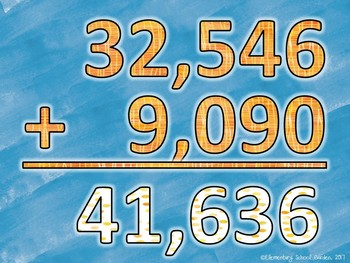# Math Movement (Math Fluency Exercise Break) - ADDITIONResource Type
File Type

Zip

(36 MB|108 pages)
Standards
Also included in:
1. Math Movement blends math fluency with an exercising and movement brain break in the classroom. This is a BUNDLE of my first 5 packs: Addition, Subtraction, Multiplication, Division, and Word Problems.I use Math Movement once or twice a week at the beginning of our math block. It helps us transiti
\$18.75
\$16.88
Save \$1.87
• Product Description
• StandardsNEW
Math Movement blends math fluency with an exercising and movement brain break in the classroom. This particular pack focuses on ADDITION!

I use Math Movement once or twice a week at the beginning of our math block. It helps us transition from the previous subject while also becoming more fluent at previously learned skills and procedures.

This pack includes:
- Teacher Directions
- 6 leveled Math Movement PDF files (all focused on Addition)
- Each file contains 6 rounds of math problems, answers (for students to check their work), and an exercise to be completed before moving on to the next problem.

Levels Include:
- Level 1: 2 and 3-digit Addition NO REGROUPING
- Level 2: 4-digit Addition REGROUPING
- Level 3: 5-digit Addition REGROUPING
- Level 4: 5-digit Addition REGROUPING
- Level 6: Finding Perimeter

Math Movement only takes up a few minutes of your day but is sure to leave a smile on your students’ faces. After a quick round of Math Movement students will be ready and rearing to move on to your daily math lesson.
Solve multistep word problems posed with whole numbers and having whole-number answers using the four operations, including problems in which remainders must be interpreted. Represent these problems using equations with a letter standing for the unknown quantity. Assess the reasonableness of answers using mental computation and estimation strategies including rounding.
Multiply or divide to solve word problems involving multiplicative comparison, e.g., by using drawings and equations with a symbol for the unknown number to represent the problem, distinguishing multiplicative comparison from additive comparison.
Interpret a multiplication equation as a comparison, e.g., interpret 35 = 5 × 7 as a statement that 35 is 5 times as many as 7 and 7 times as many as 5. Represent verbal statements of multiplicative comparisons as multiplication equations.
Find whole-number quotients of whole numbers with up to four-digit dividends and two-digit divisors, using strategies based on place value, the properties of operations, and/or the relationship between multiplication and division. Illustrate and explain the calculation by using equations, rectangular arrays, and/or area models.
Fluently multiply multi-digit whole numbers using the standard algorithm.
Total Pages
108 pages
Included
Teaching Duration
1 month
Report this Resource to TpT
Reported resources will be reviewed by our team. Report this resource to let us know if this resource violates TpT’s content guidelines.